Courses

# Shear Force and Bending Moment Civil Engineering (CE) Notes | EduRev

## Civil Engineering (CE) : Shear Force and Bending Moment Civil Engineering (CE) Notes | EduRev

The document Shear Force and Bending Moment Civil Engineering (CE) Notes | EduRev is a part of the Civil Engineering (CE) Course Civil Engineering SSC JE (Technical).
All you need of Civil Engineering (CE) at this link: Civil Engineering (CE)

Shear Force and Bending Moment

SHEAR FORCE AND BENDING MOMENT
1. SHEAR FORCE at the cross- section of a beam may be defined as the unbalanced vertical force to the right or  left of the section.

2. BENDING MOMENT at the cross- section of a beam may be defined as the algebraic sum of the moment of the forces, to the right or left of the section.

3. BEAM is a structural number subjected to transverse loads only.

4. BEAMS can be classified as :
(i) Cantilever
(ii) Simply supported
(iii) Overhanging
(iv) Rigidly fixed OR Built- in
(v) Continuous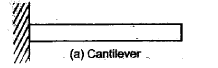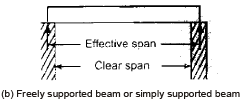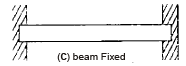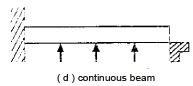5. Shear force and bending moment diagrams: Sign Convention:

(i) Shear force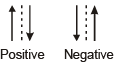(ii) Bending moment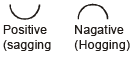SFD and BMD for cantilever beams: (i) Cantilever of length l carrying a concentrated load W at the free end
S= + W

M= – Wx

Mmax = – WL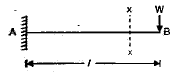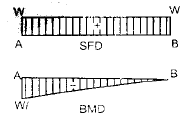(ii) Cantilever of length l carrying a uniformly distributed load of 'w' per unit run over the  whole length
Sx = + wx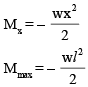Smax = + wl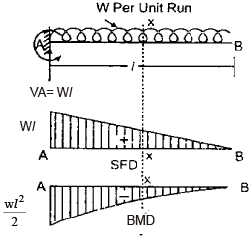(iii) Cantilever of length l carrying a uniformly distributed load of 'w' per unit run over the whole length and a concentrated load W at the free end Sx = wx + W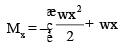Smax = wl + W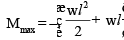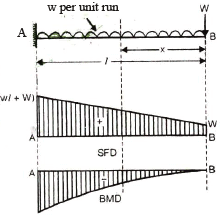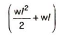(iv) Cantilever of length l carrying a uniformly distributed load of 'w' per unit run for a distance 'a' form free end form D to B,

Sx = + wx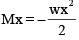form A Sx = + wa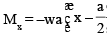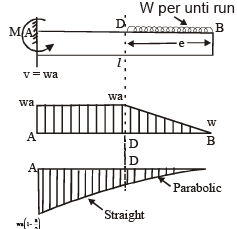(v) Cantilever of length 'l' carrying a load whose intensity varies uniformly from zero at free end to 'w' per unit run at the fixed end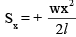= area of load diagram between X and B,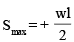Mx = Moment of load acting on XB about X = area of the load diagram between X and B × distance of centroid of this diagram form X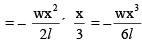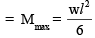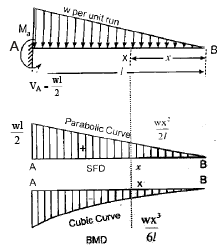(vi) Cantilever carrying a load whose  intensity varies uniformly form zero at the fixed end to w per unit run at the free  end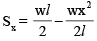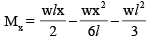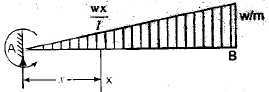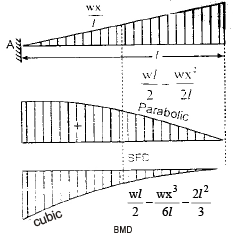SFD and  BMD for simply supported beams: (i) Simply supported beam of span l carrying a concentrated load at mid span

Sx = +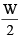(between AC)
Sx = –(between CB)
Mx = +x (between CB)
Mx = +x (form A to C) (at a distance 'X' form A)
Mmax = Mc =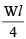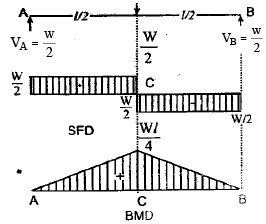(ii) Simply supported beam carrying a concentrated load placed eccentrically on the span

Sx  =  +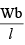(form A to D)
= –(form D to B)
Mx = +x (form A to D) at a distance 'x' form  A
Mmax = MD =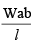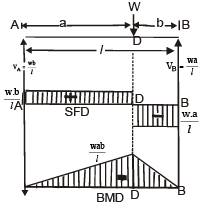NOTE: Maximum B.M. occurs where S.F. changes its sign.

(iii) Simply supported beam carrying a uniformly distributed load of w per unit run over the whole span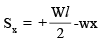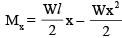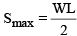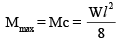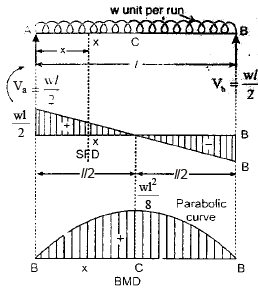(iv) Simply supported beam carrying a load whose intensity varies uniformly from zero at each end to 'w' per unit run at the mid span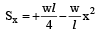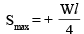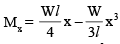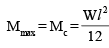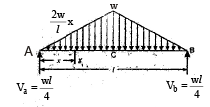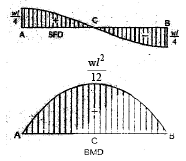(v) Simply supported  beam carrying a load whose intensity varies uniformly from zero at one end to 'w' per unit run at the other end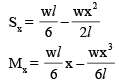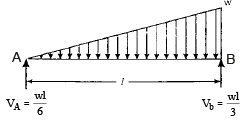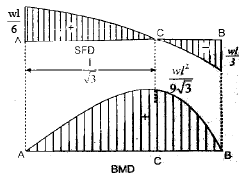Mmax B.M. occurs at x =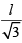form end A
Mmax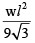SFD and BMD for simply supported beams with overhang: Simply supported beam with equal overhangs and carrying a uniformly distributed load of 'w' per unit run over the whole length S.F. at any section in EA at a distance x form E,
Sx = –wx at any section in A.B,

Sx = (w/2)(l + 2a )– wx

B.M. at any section in EA,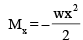at any section in AB.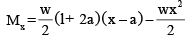at x = 'a' and 'a + l' i.e., at A & B,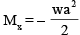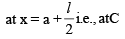Mc = (w/2)( l2 - 4a2 )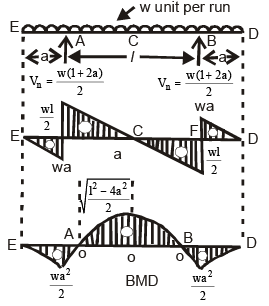Case (a) :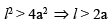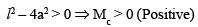∴  B.M.D. will be as shown in figure above of contraflexure O1 & O2 are at a distance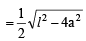form   centre.
Thus  distance between point of contraflexure OO=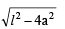Thus  distance between point of contraflexure

Case (b) :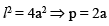B.M at C = Mc = 0 The beam will be subject to only  hogging moments.
Points of contraflexure O1 & O2 will coincide with C.
B.M.D will be as shown in figure (a)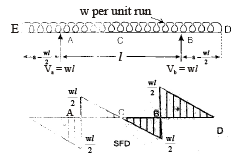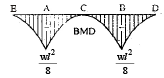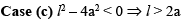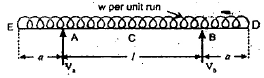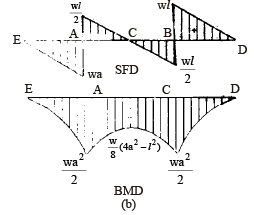Mc is negative ,since l2 < 4a2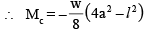B. M.  will be zero only at ends A and D and at all other sections B.M. will be of hogging type B.M. and S.F due to a couple

Case (a): Cantilever There will be no shear force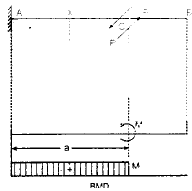Case (b): Simple supported
Shear force is constant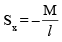B.M., Mx = –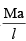(left of C)
= +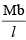(right of C)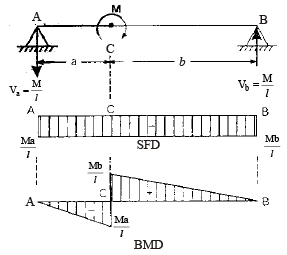Offer running on EduRev: Apply code STAYHOME200 to get INR 200 off on our premium plan EduRev Infinity!

## Civil Engineering SSC JE (Technical)

109 docs|50 tests

,

,

,

,

,

,

,

,

,

,

,

,

,

,

,

,

,

,

,

,

,

;